2020/08/30

## 一、前言

`你以为考你个数据结构是要造火箭？`

🚕汽车75马力就够奔跑了，那你怎么还想要2.0涡轮+9AT呢？大桥两边的护栏你每次走的时候都会去摸吗？那怎么没有护栏的大桥你不敢上呢？

## 二、面试题

`谢飞机`，ArrayList资料看了吧？嗯，那行问问你哈🦀

`问`：ArrayList和LinkedList，都用在什么场景呢？

`答`：啊，这我知道了。ArrayList是基于数组实现、LinkedList是基于双向链表实现，所以基于数据结构的不同，遍历和查找多的情况下用ArrayList、插入和删除频繁的情况下用LinkedList。

`问`：嗯，那LinkedList的插入效率一定比ArrayList好吗？

`答`：对，好！## 三、数据结构

`Linked + List = 链表 + 列表 = LinkedList = 链表列表`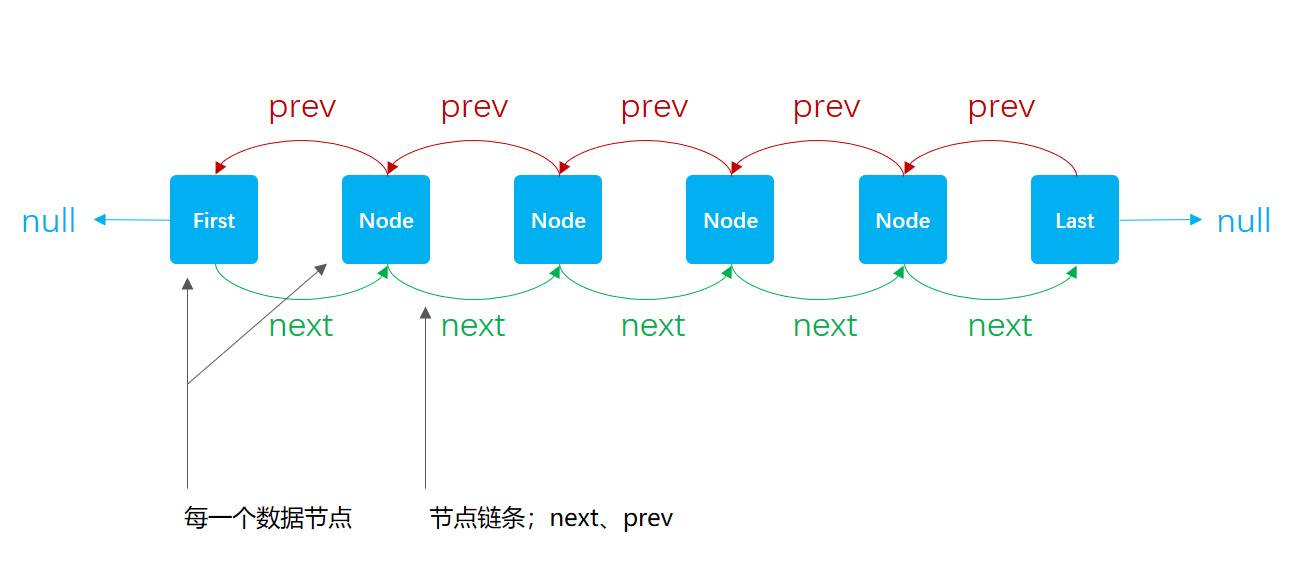## 四、源码分析

### 1. 初始化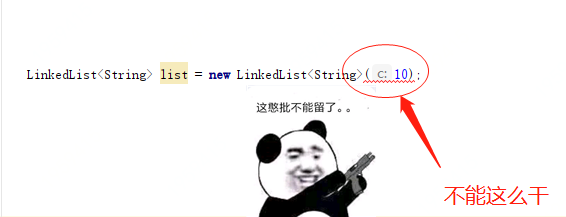``````@Test
public void test_init() {
// 初始化方式；普通方式
System.out.println(list01);

// 初始化方式；Arrays.asList
System.out.println(list02);

// 初始化方式；内部类
\\};
System.out.println(list03);

// 初始化方式；Collections.nCopies
System.out.println(list04);
}

// 测试结果

[a, b, c]
[a, b, c]
[a, b, c]
[0, 0, 0, 0, 0, 0, 0, 0, 0, 0]

Process finished with exit code 0
``````
• 这些方式都可以初始化操作，按需选择即可。

### 2. 插入

LinkedList的插入方法比较多，List中接口中默认提供的是add，也可以指定位置插入。但在LinkedList中还提供了头插`addFirst`和尾插`addLast`

#### 2.1 头插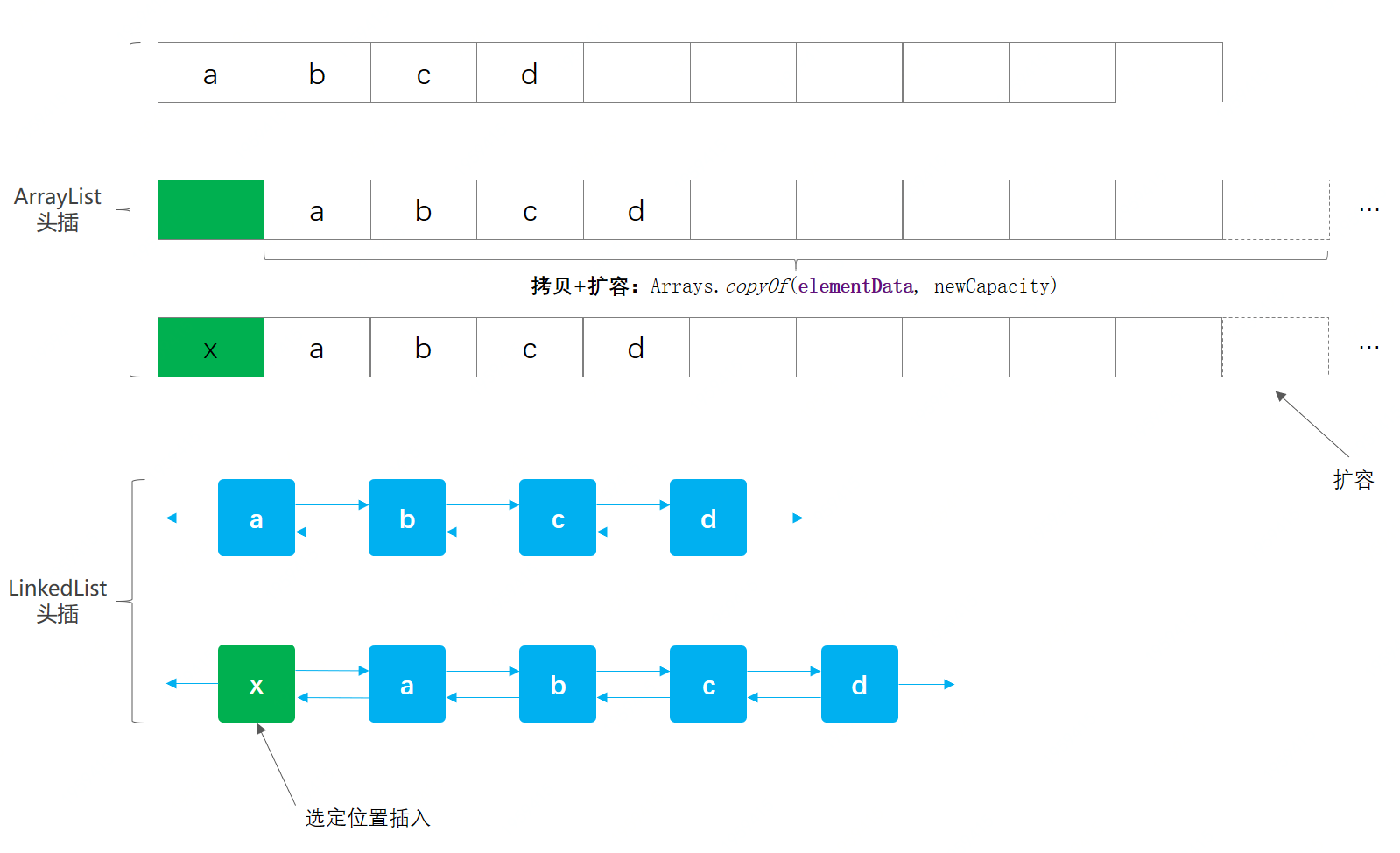1. ArrayList 头插时，需要把数组元素通过`Arrays.copyOf`的方式把数组元素移位，如果容量不足还需要扩容。
##### 2.1.1 源码

``````private void linkFirst(E e) {
final Node<E> f = first;
final Node<E> newNode = new Node<>(null, e, f);
first = newNode;
if (f == null)
last = newNode;
else
f.prev = newNode;
size++;
modCount++;
}
``````
• first，首节点会一直被记录，这样就非常方便头插。
• 插入时候会创建新的节点元素，`new Node<>(null, e, f)`，紧接着把新的头元素赋值给first。
• 之后判断f节点是否存在，不存在则把头插节点作为最后一个节点、存在则用f节点的上一个链条prev链接。
• 最后记录size大小、和元素数量modCount。modCount用在遍历时做校验，modCount != expectedModCount
##### 2.1.2 验证

``````@Test
ArrayList<Integer> list = new ArrayList<Integer>();
long startTime = System.currentTimeMillis();
for (int i = 0; i < 10000000; i++) {
}
System.out.println("耗时：" + (System.currentTimeMillis() - startTime));
}

@Test
long startTime = System.currentTimeMillis();
for (int i = 0; i < 10000000; i++) {
}
System.out.println("耗时：" + (System.currentTimeMillis() - startTime));
}
``````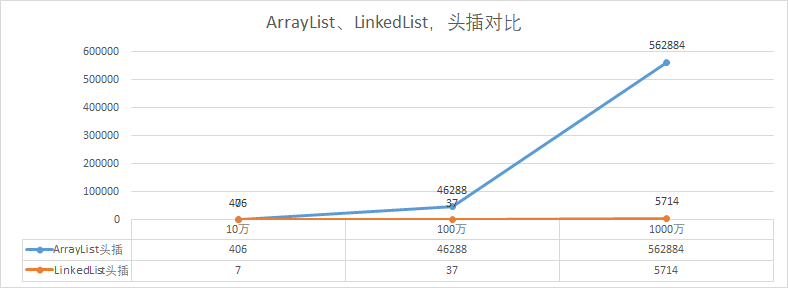• 这里我们分别验证，10万、100万、1000万的数据量，在头插时的一个耗时情况。

#### 2.2 尾插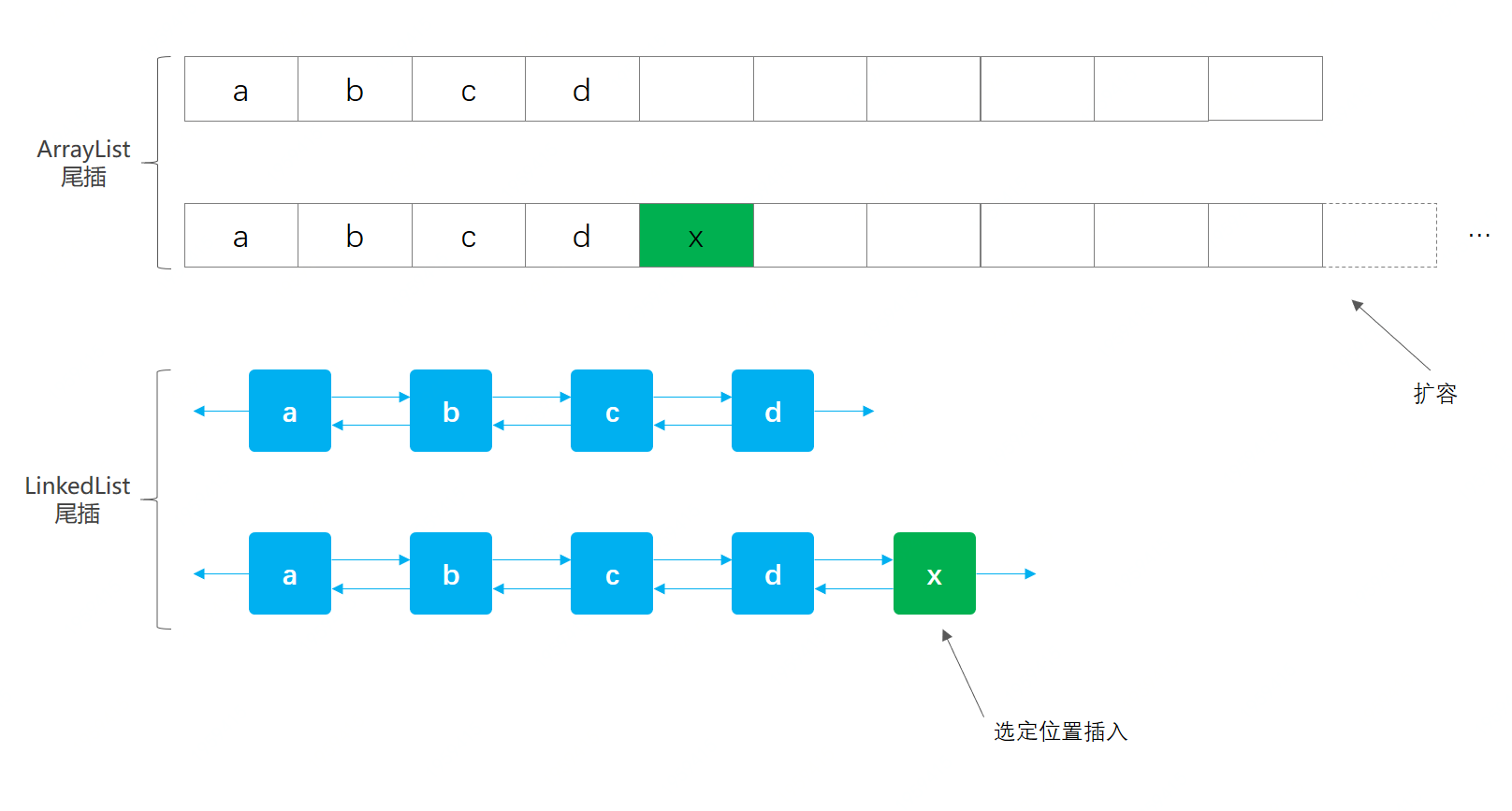1. ArrayList 尾插时，是不需要数据位移的，比较耗时的是数据的扩容时，需要拷贝迁移。
##### 2.2.1 源码

``````void linkLast(E e) {
final Node<E> l = last;
final Node<E> newNode = new Node<>(l, e, null);
last = newNode;
if (l == null)
first = newNode;
else
l.next = newNode;
size++;
modCount++;
}
``````
• 与头插代码相比几乎没有什么区别，只是first换成last
• 耗时点只是在创建节点上，`Node<E>`
##### 2.2.2 验证

``````@Test
ArrayList<Integer> list = new ArrayList<Integer>();
long startTime = System.currentTimeMillis();
for (int i = 0; i < 10000000; i++) {
}
System.out.println("耗时：" + (System.currentTimeMillis() - startTime));
}

@Test
long startTime = System.currentTimeMillis();
for (int i = 0; i < 1000000; i++) {
}
System.out.println("耗时：" + (System.currentTimeMillis() - startTime));
}
``````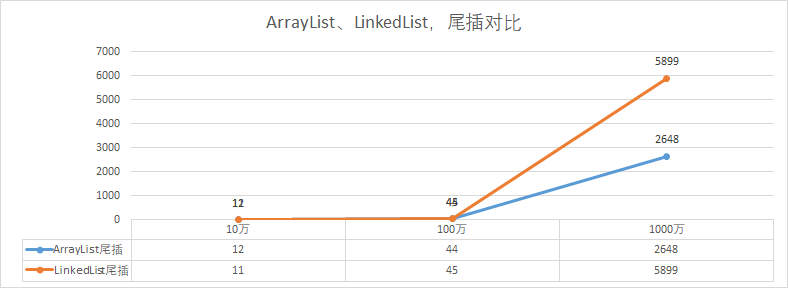• 这里我们分别验证，10万、100万、1000万的数据量，在尾插时的一个耗时情况。

#### 2.3 中间插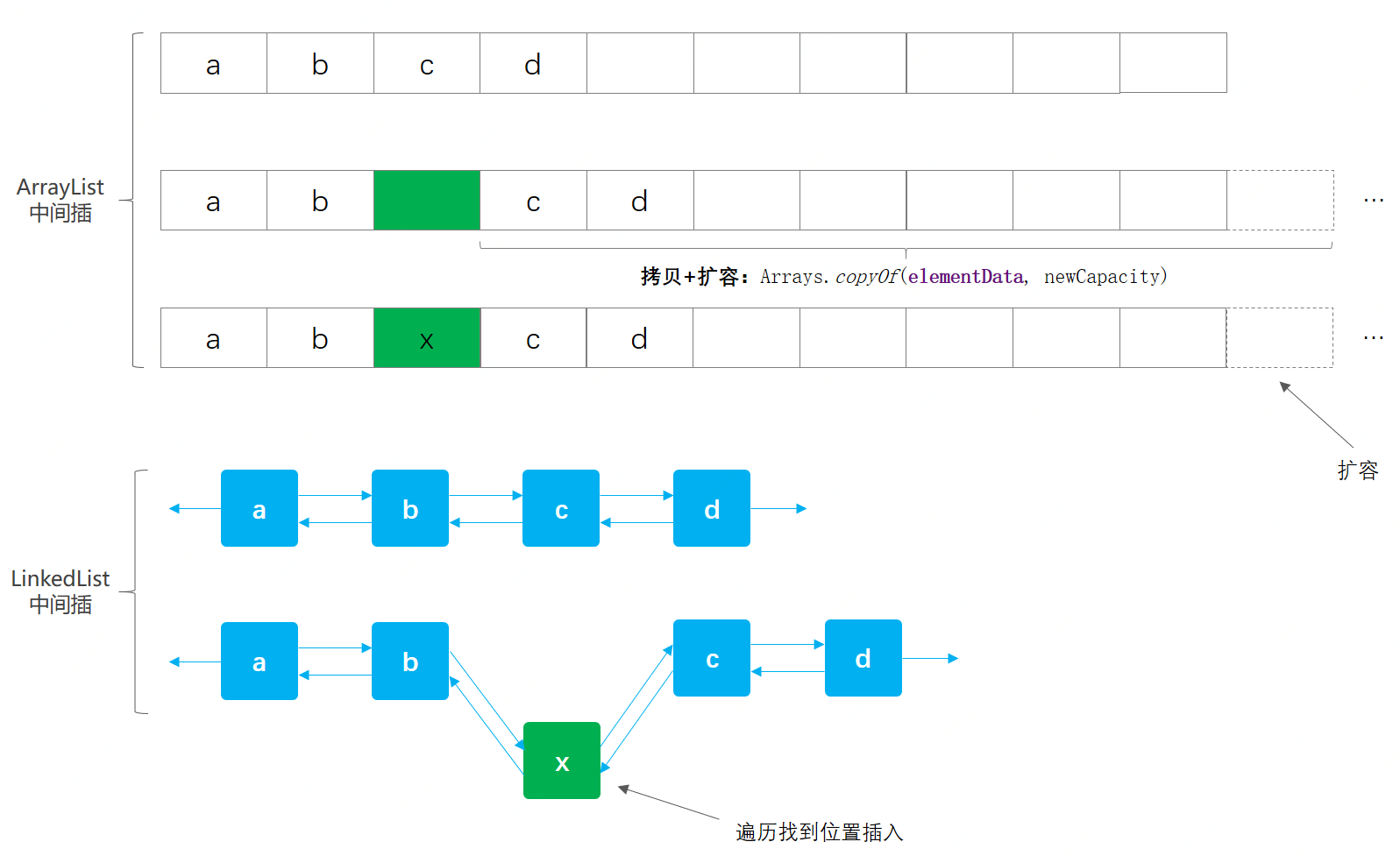1. ArrayList 中间插入，首先我们知道他的定位时间复杂度是O(1)，比较耗时的点在于数据迁移和容量不足的时候扩容。
##### 2.3.1 源码

``````public void add(int index, E element) {
checkPositionIndex(index);
if (index == size)
else
}
``````

``````Node<E> node(int index) {
// assert isElementIndex(index);
if (index < (size >> 1)) {
Node<E> x = first;
for (int i = 0; i < index; i++)
x = x.next;
return x;
} else {
Node<E> x = last;
for (int i = size - 1; i > index; i--)
x = x.prev;
return x;
}
}
``````
• `size >> 1`，这部分的代码判断元素位置在左半区间，还是右半区间，在进行循环查找。

``````void linkBefore(E e, Node<E> succ) {
// assert succ != null;
final Node<E> pred = succ.prev;
final Node<E> newNode = new Node<>(pred, e, succ);
succ.prev = newNode;
if (pred == null)
first = newNode;
else
pred.next = newNode;
size++;
modCount++;
}
``````
• 找到指定位置插入的过程就比较简单了，与头插、尾插，相差不大。
• 整个过程可以看到，插入中比较耗时的点会在遍历寻找插入位置上。
##### 2.3.2 验证

``````@Test
ArrayList<Integer> list = new ArrayList<Integer>();
long startTime = System.currentTimeMillis();
for (int i = 0; i < 10000000; i++) {
}
System.out.println("耗时：" + (System.currentTimeMillis() - startTime));
}

@Test
long startTime = System.currentTimeMillis();
for (int i = 0; i < 1000000; i++) {
}
System.out.println("耗时：" + (System.currentTimeMillis() - startTime));
}
``````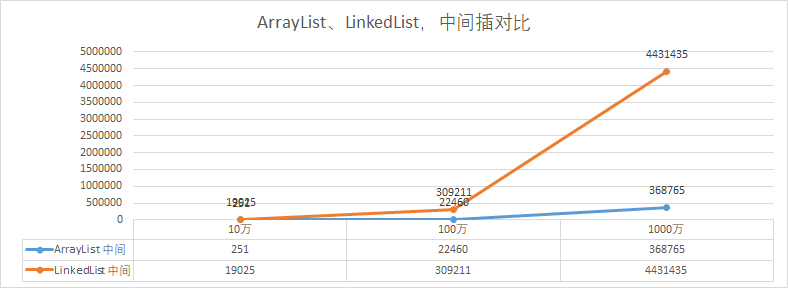• 这里我们分别验证，10万、100万、1000万的数据量，在中间插时的一个耗时情况。

### 3. 删除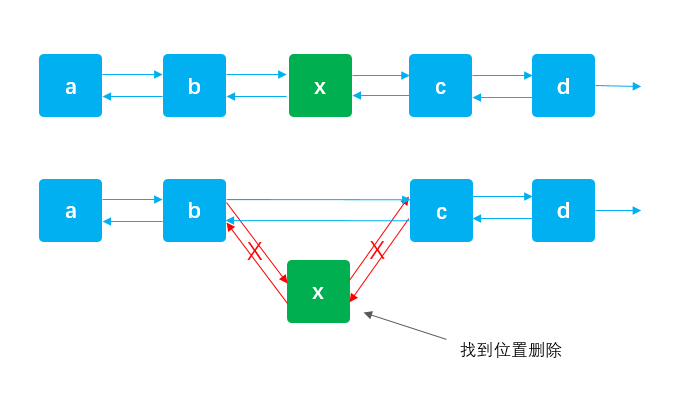• 确定出要删除的元素x，把前后的链接进行替换。
• 如果是删除首尾元素，操作起来会更加容易，这也就是为什么说插入和删除快。但中间位置删除，需要遍历找到对应位置。

#### 3.1 删除操作方法

1 list.remove(); 与removeFirst()一致
2 list.remove(1); 删除Idx=1的位置元素节点，需要遍历定位
3 list.remove(“a”); 删除元素=”a”的节点，需要遍历定位
4 list.removeFirst(); 删除首位节点
5 list.removeLast(); 删除结尾节点
6 list.removeAll(Arrays.asList(“a”, “b”)); 按照集合批量删除，底层是Iterator删除

``````@Test
public void test_remove() {

list.remove();
list.remove(1);
list.remove("a");
list.removeFirst();
list.removeLast();
list.removeAll(Arrays.asList("a", "b"));
}
``````

#### 3.2 源码

list.remove(“a”);

``````public boolean remove(Object o) {
if (o == null) {
for (Node<E> x = first; x != null; x = x.next) {
if (x.item == null) {
return true;
}
}
} else {
for (Node<E> x = first; x != null; x = x.next) {
if (o.equals(x.item)) {
return true;
}
}
}
return false;
}
``````
• 这一部分是元素定位，和` unlink(x)`解链。循环查找对应的元素，这部分没有什么难点。

``````E unlink(Node<E> x) {
// assert x != null;
final E element = x.item;
final Node<E> next = x.next;
final Node<E> prev = x.prev;

if (prev == null) {
first = next;
} else {
prev.next = next;
x.prev = null;
}

if (next == null) {
last = prev;
} else {
next.prev = prev;
x.next = null;
}

x.item = null;
size--;
modCount++;
return element;
}
``````

1. 获取待删除节点的信息；元素item、元素下一个节点next、元素上一个节点prev。
2. 如果上个节点为空则把待删除元素的下一个节点赋值给首节点，否则把待删除节点的下一个节点，赋值给待删除节点的上一个节点的子节点。
3. 同样待删除节点的下一个节点next，也执行2步骤同样操作。
4. 最后是把删除节点设置为null，并扣减size和modeCount数量。

### 4. 遍历

``````int xx = 0;
@Before
public void init() {
for (int i = 0; i < 10000000; i++) {
}
}
``````

#### 4.1 普通for循环

``````@Test
long startTime = System.currentTimeMillis();
for (int i = 0; i < list.size(); i++) {
xx += list.get(i);
}
System.out.println("耗时：" + (System.currentTimeMillis() - startTime));
}
``````

#### 4.2 增强for循环

``````@Test
long startTime = System.currentTimeMillis();
for (Integer itr : list) {
xx += itr;
}
System.out.println("耗时：" + (System.currentTimeMillis() - startTime));
}
``````

#### 4.3 Iterator遍历

``````@Test
long startTime = System.currentTimeMillis();
Iterator<Integer> iterator = list.iterator();
while (iterator.hasNext()) {
Integer next = iterator.next();
xx += next;
}
System.out.println("耗时：" + (System.currentTimeMillis() - startTime))
}
``````

#### 4.4 forEach循环

``````@Test
long startTime = System.currentTimeMillis();
list.forEach(integer -> {
xx += integer;
});
System.out.println("耗时：" + (System.currentTimeMillis() - startTime));
}
``````

#### 4.5 stream(流)

``````@Test
long startTime = System.currentTimeMillis();
list.stream().forEach(integer -> {
xx += integer;
});
System.out.println("耗时：" + (System.currentTimeMillis() - startTime));
}
``````

## 五、总结

• ArrayList与LinkedList都有自己的使用场景，如果你不能很好的确定，那么就使用ArrayList。但如果你能确定你会在集合的首位有大量的插入、删除以及获取操作，那么可以使用LinkedList，因为它都有相应的方法；`addFirst``addLast``removeFirst``removeLast``getFirst``getLast`，这些操作的时间复杂度都是O(1)，非常高效。